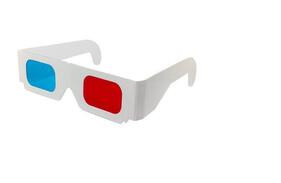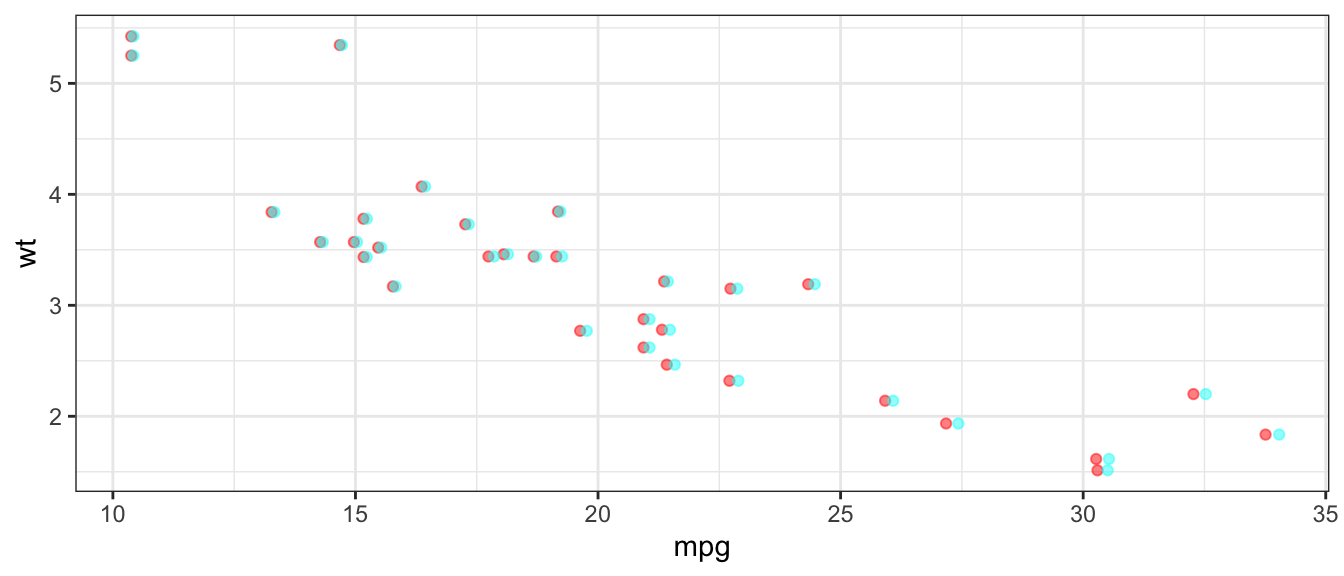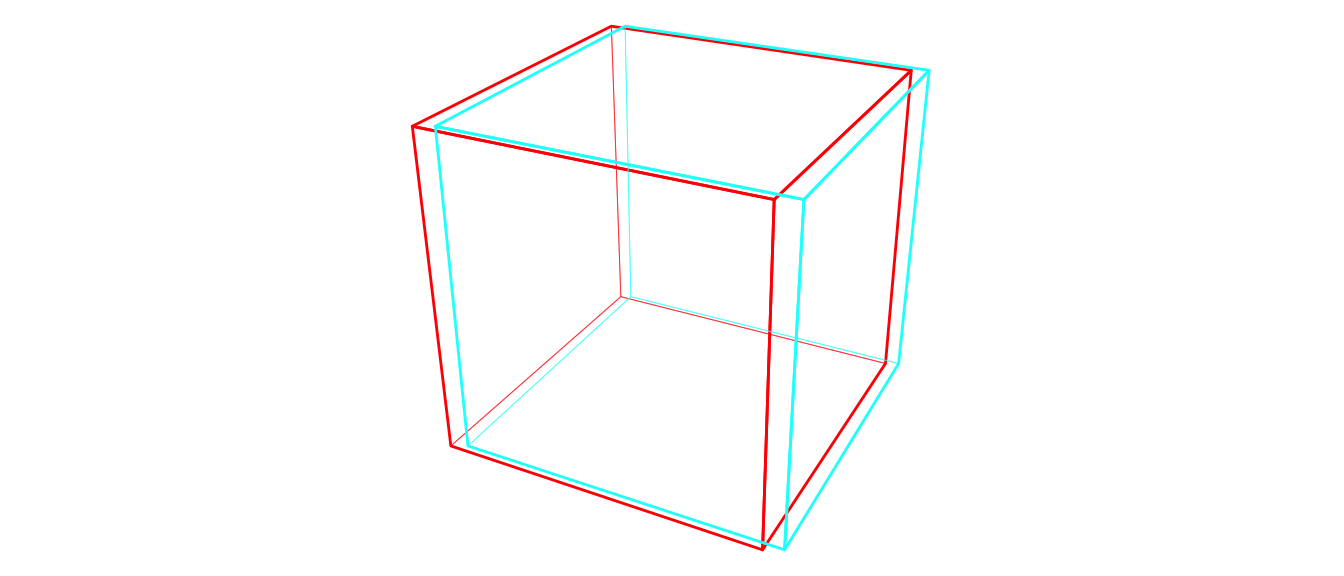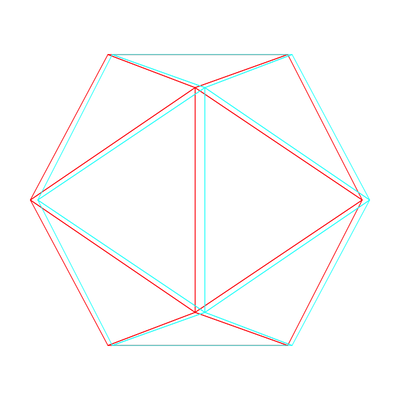# Red/Blue Analglyphs with ggplot2 and ggthreed

## ggthreed v0.1.2

ggthreed now includes a stat_anaglyph() for creating red/blue anaglyph plots.

## Installation

You can install from github

# install.packages("devtools")
devtools::install_github("coolbutuseless/threed")
devtools::install_github("coolbutuseless/ggthreed")

# Red/blue anaglyphs with stat_anaglyph()## Usage

• Works with point, line and polygon geoms. Although “works” may be a strong term - the 3d effect is very weak even if you tweak all the parameters
• It might work with other geoms, but no guarantees that it produces anything worth looking at.
• parameters:
• zoffset, zscale - for determining how z aesthetic influences position
• red, blue - hex colours to use for red/blue
• switch - switch position of the colours

## Example red/blue anaglyphs with points.

ggplot(mtcars) +
geom_point(aes(mpg, y = wt, z = disp), stat = 'anaglyph', alpha = 0.5, zscale = 10) +
theme_bw()## Example of combined 3d projection and anaglyph

library(threed)

camera_to_world <- look_at_matrix(eye = c(1.5, 1.75, 3), at = c(0, 0, 0))

obj <- threed::mesh3dobj\$cube %>%
transform_by(invert_matrix(camera_to_world)) %>%
translate_by(c(0, 0, -3)) %>%
perspective_projection()

ggplot(obj, aes(x, y, z = z, group = element_id)) +
geom_polygon(fill = NA, colour='black', aes(size = hidden), stat = 'anaglyph',
zscale = 0.05, zoffset = -1.4, zinvert = FALSE) +
scale_linetype_manual(values = c('TRUE' = "FF", 'FALSE' = 'solid')) +
scale_size_manual(values = c('TRUE' = 0.1, 'FALSE' = 0.5)) +
theme_void() +
theme(legend.position = 'none') +
coord_equal()## Animated Icosahedron

See vignette('animated-anaglyph', package='ggthreed')